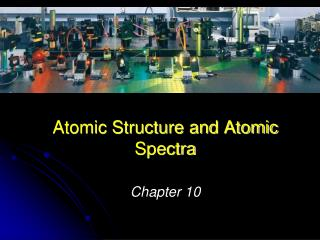Download PresentationAtomic Structure and Atomic Spectra

# Atomic Structure and Atomic Spectra - PowerPoint PPT Presentation

Atomic Structure and Atomic Spectra. Chapter 10. Structures of many-electron atoms. Because of electron correlation, no simple analytical expression for orbitals is possible Therefore ψ (r 1 , r 2 , ….) can be expressed as ψ (r 1 ) ψ (r 2 )… Called the orbital approximationI am the owner, or an agent authorized to act on behalf of the owner, of the copyrighted work described.
Download Presentation## Atomic Structure and Atomic Spectra

Download Policy: Content on the Website is provided to you AS IS for your information and personal use and may not be sold / licensed / shared on other websites without getting consent from its author.While downloading, if for some reason you are not able to download a presentation, the publisher may have deleted the file from their server.

- - - - - - - - - - - - - - - - - - - - - - - - - - E N D - - - - - - - - - - - - - - - - - - - - - - - - - -
Presentation Transcript
1. Atomic Structure and Atomic Spectra Chapter 10

2. Structures of many-electron atoms • Because of electron correlation, no simple analytical • expression for orbitals is possible • Therefore ψ(r1, r2, ….) can be expressed as ψ(r1)ψ(r2)… • Called the orbital approximation • Individual hydrogenic orbitals modified by presence of • other electrons

3. Structures of many-electron atoms Pauli exclusion principle– no more than two electrons may occupy an atomic orbital, and if so, must be of opposite spin

4. Structures of many-electron atoms • In many-electron atoms, subshells are not • degenerate. Why? • Shielding and penetration

5. Fig 10.19 Shielding and effective nuclear charge, Zeff • Shielding from core electrons • reduces Z to Zeff • Zeff = Z – σ • where σ ≡ shielding constant

6. Fig 10.20 Penetration of 3s and 3p electrons • Shielding constant different for s and p electrons • s-electron has greater • penetration and is bound more • tightly bound • Result: s < p < d < f

7. Structure of many-electron atoms • In many-electron atoms, subshells are not • degenerate. Why? • Shielding and penetration • The building-up principle (Aufbau) • Mnemonic:

8. Order of orbitals (filling) in a many-electron atom 1s < 2s < 2p < 3s < 3p < 4s < 3d < 4p < 5s < 4d < 5p < 6s

9. ? ? “Fill up” electrons in lowest energy orbitals (Aufbau principle) Li 3 electrons Be 4 electrons B 5 electrons C 6 electrons B 1s22s22p1 Be 1s22s2 Li 1s22s1 H 1 electron He 2 electrons He 1s2 H 1s1

10. Structure of many-electron atoms • In many-electron atoms, subshells are not • degenerate. Why? • Shielding and penetration • The building-up principle (Aufbau) • Mnemonic: • Hund’s rule of maximum multiplicity • Results from spin correlation

11. The most stable arrangement of electrons in subshells is the one with the greatest number of parallel spins (Hund’s rule). C 6 electrons N 7 electrons O 8 electrons F 9 electrons Ne 10 electrons Ne 1s22s22p6 C 1s22s22p2 N 1s22s22p3 O 1s22s22p4 F 1s22s22p5

12. Fig 10.21 Electron-electron repulsions in Sc atom Reduced repulsions with configuration [Ar] 3d1 4s2 If configuration was [Ar] 3d2 4s1

13. I1+ X(g) X+(g)+ e- I2+ X+(g) X2+(g)+ e- I3+ X2+(g) X3+(g)+ e- Ionization energy (I) - minimum energy (kJ/mol) required to remove an electron from a gaseous atom in its ground state I1 first ionization energy I2 second ionization energy I3 third ionization energy I1 < I2 < I3

14. Mg → Mg+ + e− I1 = 738 kJ/mol For Mg2+ 1s22s22p6 Mg+→ Mg2+ + e− I2 = 1451 kJ/mol Mg2+→ Mg3+ + e− I3 = 7733 kJ/mol

15. Fig 10.22 First ionization energies N [He] 2s2 2p3 I1 = 1400 kJ/mol O [He] 2s2 2p4 I1 = 1314 kJ/mol

16. Spectra of complex atoms • Energy levels not solely given by energies • of orbitals • Electrons interact and make contributions to E

17. Fig 10.18 Vector model for paired-spin electrons Multiplicity = (2S + 1) = (2·0 + 1) = 1 Singlet state Spins are perfectly antiparallel

18. Fig 10.24 Vector model for parallel-spin electrons Three ways to obtain nonzero spin Multiplicity = (2S + 1) = (2·1 + 1) = 3 Triplet state Spins are partially parallel

19. Fig 10.25 Grotrian diagram for helium Singlet – triplet transitions are forbidden

20. Fig 10.26 Orbital and spin angular momenta Spin-orbit coupling Magnetogyric ratio

21. Fig 10.27(a) Parallel magnetic momenta Total angular momentum (j) = orbital (l) + spin (s) e.g., for l = 0 → j = ½

22. Fig 10.27(b) Opposed magnetic momenta Total angular momentum (j) = orbital (l) + spin (s) e.g., for l = 0 → j = ½ for l = 1 → j = 3/2, ½

23. Fig 10.27 Parallel and opposed magnetic momenta Result: For l > 0, spin-orbit coupling splits a configuration into levels

24. Fig 13.30 Spin-orbit coupling of a d-electron (l = 1) j = l + 1/2 j = l - 1/2

25. Energy levels due to spin-orbit coupling • Strength of spin-orbit coupling depends on • relative orientations of spin and orbital • angular momenta (= total angular momentum) • Total angular momentum described in terms of • quantum numbers: j and mj • Energy of level with QNs: s, l, and j • where A is the spin-orbit coupling constant El,s,j = 1/2hcA{ j(j+1) – l(l+1) – s(s+1) }